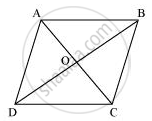Share

# Show that the Diagonals of a Parallelogram Divide It into Four Triangles of Equal Area. - CBSE Class 9 - Mathematics

ConceptTriangles on the Same Base and Between the Same Parallels

#### Question

Show that the diagonals of a parallelogram divide it into four triangles of equal area.

#### SolutionWe know that diagonals of parallelogram bisect each other.

Therefore, O is the mid-point of AC and BD.

BO is the median in ΔABC. Therefore, it will divide it into two triangles of equal areas.

∴ Area (ΔAOB) = Area (ΔBOC) ... (1)

In ΔBCD, CO is the median.

∴ Area (ΔBOC) = Area (ΔCOD) ... (2)

Similarly, Area (ΔCOD) = Area (ΔAOD) ... (3)

From equations (1), (2), and (3), we obtain

Area (ΔAOB) = Area (ΔBOC) = Area (ΔCOD) = Area (ΔAOD)

Therefore, it is evident that the diagonals of a parallelogram divide it into four triangles of equal area.

Is there an error in this question or solution?

#### APPEARS IN

NCERT Solution for Mathematics Class 9 (2018 to Current)
Chapter 9: Areas of Parallelograms and Triangles
Ex. 9.30 | Q: 3 | Page no. 162

#### Video TutorialsVIEW ALL 

Solution Show that the Diagonals of a Parallelogram Divide It into Four Triangles of Equal Area. Concept: Triangles on the Same Base and Between the Same Parallels.
S